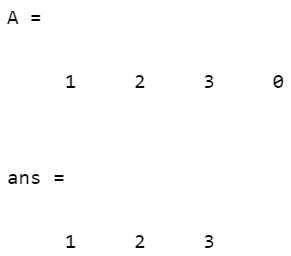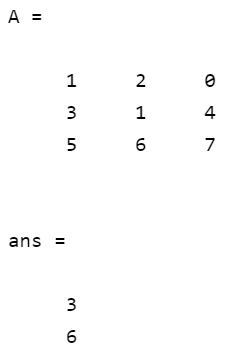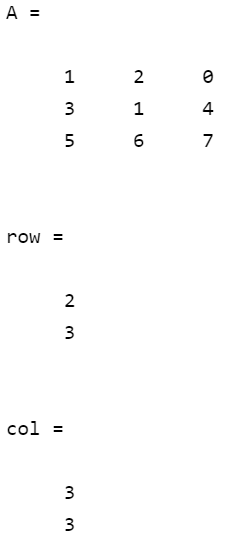# Find() function in MATLAB

• Difficulty Level : Medium
• Last Updated : 21 Apr, 2021

The find() function in MATLAB is used to find the indices and values of non-zero elements or the elements which satisfy a given condition. The relational expression can be used in conjunction with find to find the indices of elements that meet the given condition. It returns a vector that contains the linear indices.

Using liner index a multidimensional array can be accessed using a single subscript. MATLAB treats the array as a single column vector with each column appended to the bottom of the previous column.

Example:

For example consider the following 3×3 array A =

1 4 7

2 5 8

3 6 9

In this array all elements represents their linear index i.e. we can reference A(1,2) with A(4).

Syntax:

Below are various wasy to use the function:

• k = find(X): It returns the indices of all non zero elements.
• k = find(X, n): It returns the first n indices of non zero elements in X
• k = find(X, n, direction): direction can be ‘first’ or ‘last’. If direction is first, this function will return first n indices corresponding to non zero elements and if direction is last then this function will return last n indices corresponding to non zero elements
• [row, col] = find(): It is used to get row and column subscript for all non zero elements.
• [row, col, v] = find(): row and column will hold subscript for all non zero elements and v is a vector which will hold all the non zero elements.

Note: k will be of same orientation as X if X is a vector and if X is a multidimensional array then k will be a column vector which will hold linear indices.

Example 1: Below code will return the indices of non-zero elements in a 1-D array.

## MATLAB

 `% Defining array``A = [1 2 3 0]`` ` `% Getting indices of non zero elements``find(A)`

Output:Example 2: Below code will return the first 2 indices of elements where the element will be greater than 3.

## MATLAB

 `% Defining array``A = [1 2 0; 3 1 4; 5 6 7]`` ` `% Getting first 2 indices``find(A>3, 2)`

Output:Example 3: Below code will return the last 2 row and column indices of elements that are greater than 3.

## MATLAB

 `% Defining array``A = [1 2 0; 3 1 4; 5 6 7]`` ` `% Getting row and column ``[row, col] = find(A>3, 2, ``'last'``)`

Output:So, A(2, 3) and A(3, 3) are the last elements that are greater than 3. We got (2, 3) and (3, 3) as output not (3,2) and (3, 3) because MATLAB treats the array as a single column vector with each column appended to the bottom of the previous column.

Example 4: Below code will return indices of all the zero elements. This code uses the negation operator (~) in conjunction with the find function.

## MATLAB

 `% Defining array``A = [1 2 3 0]`` ` `% Getting indices of zero elements``find(~A)`

Output:My Personal Notes arrow_drop_up